# An Example of Graph Convolutional Networks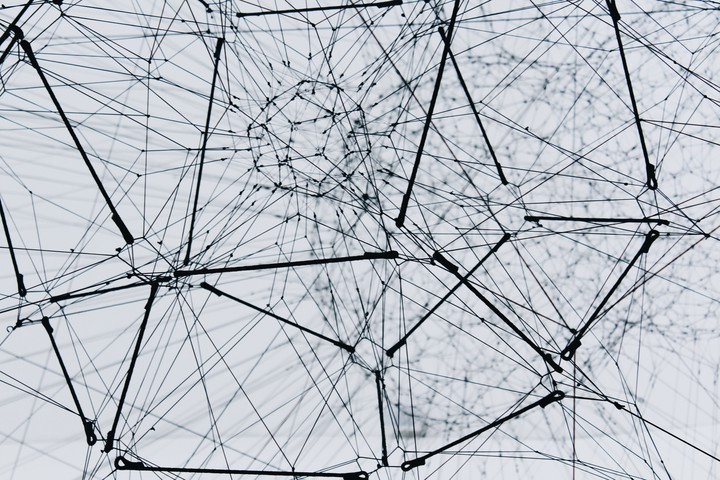Photo by Alina Grubnyak on Unsplash

## Introduction

In this post, we're going to look under the hood of Graph Convolutional Networks (GCNs). By the end, you'll understand e.g. how GCNs can discover community structure even with random parameter values before any training. We use the Deep Graph Library1 (DGL) package to run a variety of experiments in a node classification task to tease apart how graph structure, node features, and model parameters interact when computing node embeddings.

The code for reproducing this post can be found on GitHub.

## Dataset

This example will use the Citeseer dataset, which has information about academic papers and their citations. This is modeled as a graph by treating nodes as publications and (undirected) edges as citations between them. The dataset is designed for node classification tasks where the goal is to predict which of the 6 categories the publication belongs.

Nodes: 3327
Edges: 9228
Number of Classes: 6

Label Split:
Train: 120
Valid: 500
Test: 1000


Note that nodes 620-2311 are not in any of the Train/Valid/Test sets and are unused.

Each node also has a predefined feature vector with 3703 dimensions. Each column represents a word and the value is the (normalized) count of that word in the publication. If we project these features down to 2 dimensions using t-SNE and color code by the class label (Figure 1), we see some nice structure that suggests we can do a reasonable job of classification by just using the input features and ignoring the graph structure.

## Modeling

### Baselines

Before digging into graph methods, let's establish some simple baselines. Since the classes are balanced, a random guess will have accuracy of 1/6 = 17%. By training Logistic Regression and Random Forest models on just the features (i.e. no citation graph structure), accuracies of 55% and 57% were obtained, respectively.

## GCN

To compare against these baselines, I'll be using Graph Convolutional Networks2. If you're unfamiliar with this topic, you can check out my YouTube video that introduces them below. But in short, graph information is leveraged by having the nodes send their feature vectors to their connected neighbors and then an embedding is calculated for each node by aggregating these neighborhood features and passing the result through a neural network layer. In this particular use-case, the output of a GCN layer would represent the word count information of the paper in question and all its citations (passed through a non-linear neural network).

### GCN before training

Before we start training our model, let's pause to get a snapshot of how things look with randomly initialized model parameters. This will help build intuition for the different components of what makes GCNs tick.

Instead of using the word count information of each paper, let's just pass in the identity matrix. The result of this is that each node will essentially get a dedicated row in the first projection matrix. But since the neural net parameters are randomly initialized and there is no training in this case, each node will effectively be assigned to a random location as input to the GCN process. If we stack a few GCN layers together and project the final class predictions down to 2-dimensions for plotting purposes with t-SNE, we get Figure 2.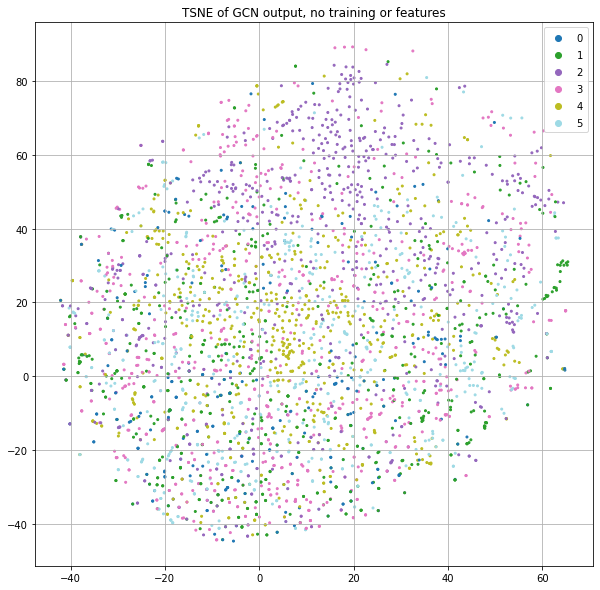Figure 2. t-SNE of GCN output without training or features. Color indicates class label.

Now wait. There are clearly little clusters of homogenous labels. If node positions are randomized by using a projection matrix with random weights and we're not using any of the input features or training, how are we already seeing community structure?!?

Let's deep-dive some points that are highly clustered to understand how this is possible. I will refer to them as “ROI nodes” (a.k.a. “Region of Interest” nodes) and Figure 3 shows which of these points we'll be deep-diving.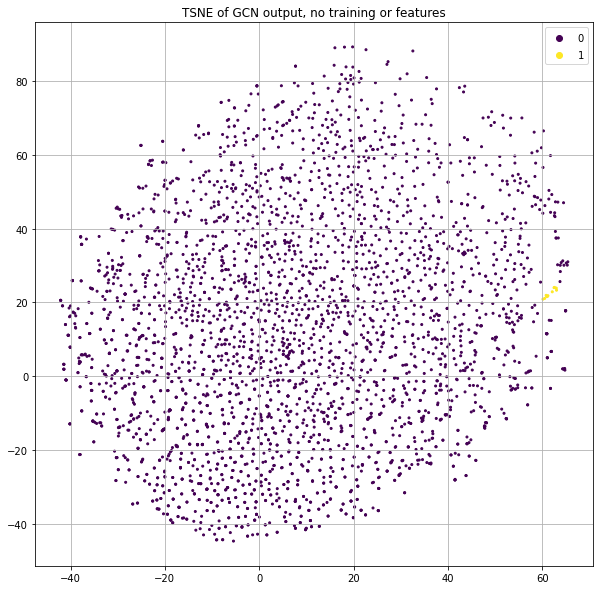Figure 3. t-SNE of GCN output without training or features. Yellow points indicate those for deep-dive analysis.

These points are nearby one another when comparing their output from our model. To understand how these nodes might relate to one another in the citation graph, let's pull all the connected nodes of each of these points to observe their connection patterns. For comparison, we'll plot some randomly sampled nodes (Figure 4).Figure 4. Graph structure of ROI nodes and their nearest neighbors in the graph (red) along with a random sample of nodes (blue). Node labels indicate class.

It looks as if all of these nodes we're deep-diving (red) are self-connected. Comparatively, when we visualize a random set of nodes (blue), there are very few inter-connections. These inter-connections are why nodes are mapped to similar embeddings by the GCN.

This can be understood by thinking of GCNs as a parameterized and differentiable approximation to the Weisfeiler-Lehman algorithm3 (see Thomas Kipf's blog on this). In short, the W-L algorithm attempts to assign unique values to each node based on its role in the graph and it can do this by summing the values of its connections and passing the result through a hash function. The GCN layer equation does essentially that, but rather than a hash function, it applies a linear projection via a learnable weight matrix $W^{(l)}$ and a non-linear activation function $\sigma$ (i.e. a dense neural network layer operates on the sum of neighbor vectors, $h_j^{(l)}$):

$$h_i^{(l+1)} = \sigma \left ( \sum_j \frac{1}{c_{ij}} h_j^{(l)}W^{(l)} \right )$$

In this case, since our projection matrix $W^{(l)}$ is randomly initialized and we're using the identity matrix as our initial features $h^{(0)}$, we effectively assign a vector of random numbers to each node $i$ as the output of the first GCN layer, $h_i^{(1)}$. Then, the process of summing up neighbors and transforming that result with additional GCN layers causes these points to get closer together since they are using many of the same neighbors in the sum process. In the edge case where a set of nodes all have the exact same neighbors, then they would be mapped to identical outputs. I.e. same inputs => same outputs.

In Figure 4, not all nodes have identical neighborhoods, but they share many of the same neighbors, so it's not a surprise that when summing those values and passing through a mapping function that they end up with similar outputs. This is how a GCN can discover community structure despite no training, features or labels. It's merely mapping things with similar graph connections to similar outputs and that's often associated with the thing you want to predict.

#### Initialize nodes with features

Now, let's add in the features rather than using the identity matrix. Figure 5 shows something similar to Figure 2, but the use of node features appears to result in significantly more structure with respect to the label.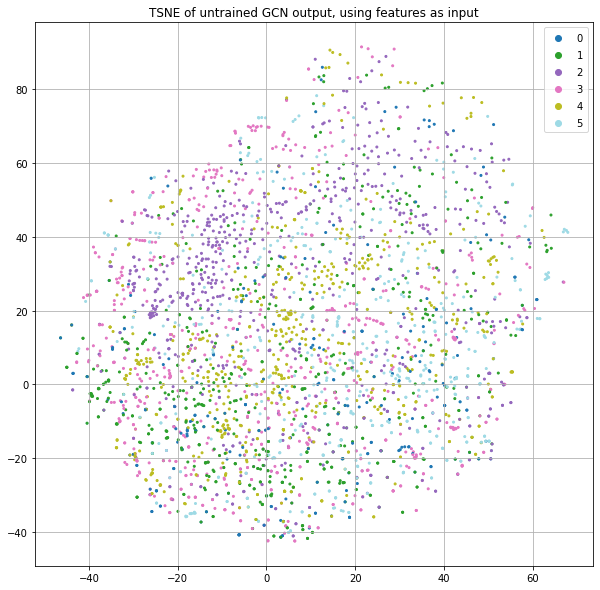Figure 5. t-SNE of untrained GCN output using features as input. Color indicates class label.

There generally seems to be more consistent class separation as well as more fine structure in the form of little clusters. This is because we're not only taking advantage of graph structure as before, but now instead of starting with random node positions (as a consequence of using the identity matrix and a randomly initialized weight matrix), we initialize nodes using their word-count vectors. This means that two publications that use the same word counts would start from the same positions rather than being placed in random positions. As we saw above when we plotted the t-SNE of just the features (Figure 1), this already encodes label information. Now there are two forces that put nodes close together: having similar words and having similar graph neighborhoods. And we still haven't trained anything.

### GCN with training

Let's go back to using the identity matrix instead of the features, but now use the training labels to optimize our GCN parameters for class prediction (Figure 6). This model results in about 50% accuracy. This is lower than the Logistic Regression (55%) and Random Forest (57%), but no node features are being used here, just the citation graph structure.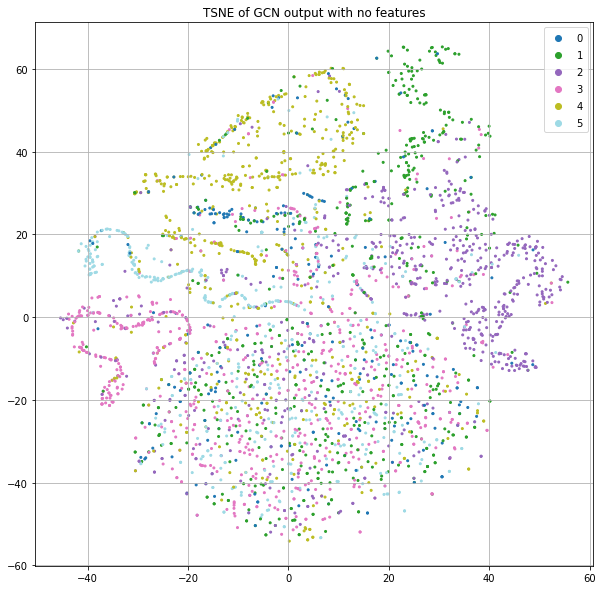Figure 6. t-SNE of GCN output using identity matrix as input. Color indicates class label.

The “legs” mostly seem to represent a coherent label that's smooth in the neighborhood, but the big blob at the bottom seems to have no structure. Let's plot the same thing, but color code by the loss value rather than class label (Figure 7).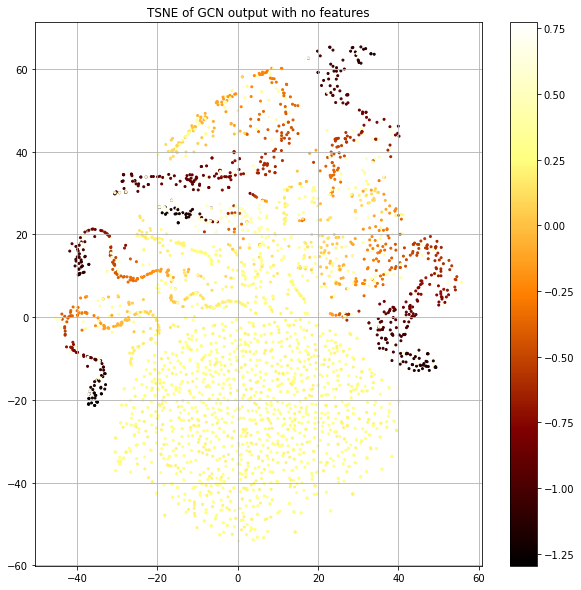Figure 7. t-SNE of GCN output using identity matrix as input. Color indicates Log of loss.

The blob has the highest loss and structured islands have lowest. This is consistent with our observation of label smoothness. Now, let's look at the number of training nodes that are present in the 1-hop neighborhood of each node (Fig. 8, left), since this provides the training signal. Let's also look at the size of each node's 1-hop neighborhood (Fig. 8, right).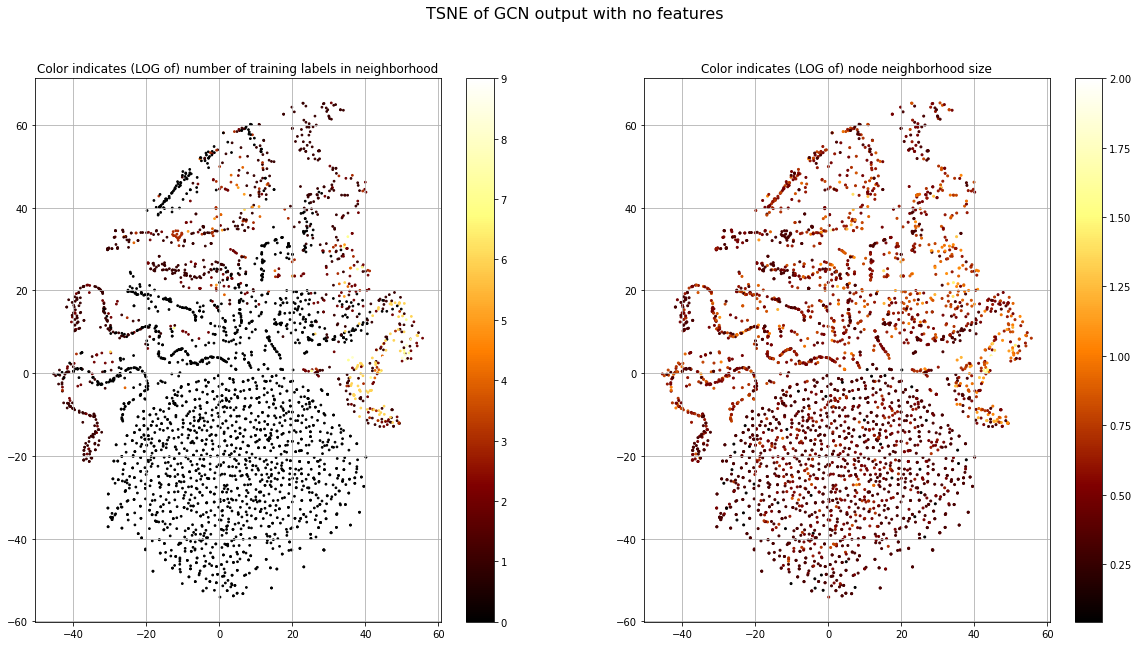Figure 8. t-SNE of GCN output using identity matrix as input. Color indicates (left) the number of training nodes in each node's 1-hop neighborhood and (right) the size of each node's 1-hop neighborhood.

This shows that there are very few training samples in the blob and other regions with high loss. This means there will not be much learning signal to update the parameters that correspond to this node. But we also see some areas with high structure in the legs that also do not have training nodes in their neighborhood. When considering the total neighborhood size we see that those with high structure, but no training node neighbors, have relatively large neighborhood size. This might indicate that these leg-nodes are structurally similar within the graph and that this information helps in grouping them.

Let's dig into a particular set of these well-structured/high-loss points to understand them more, shown in Figure 9 as yellow points.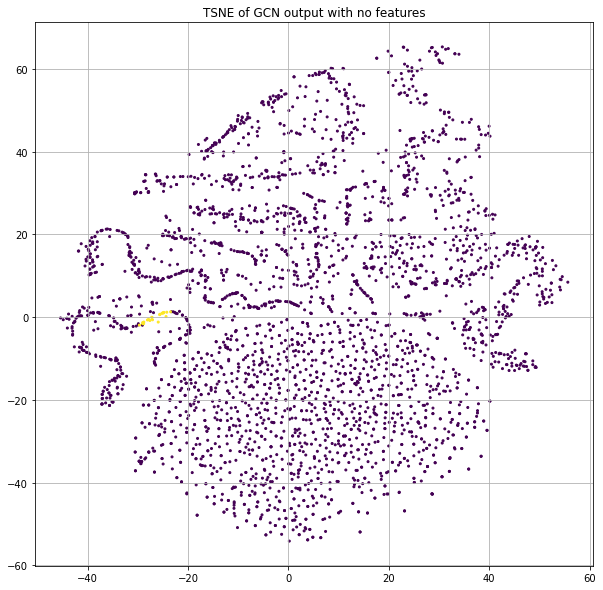Figure 9. t-SNE of GCN output using identity matrix as input. Yellow points indicate Region of Interest (ROI)

As before, we pull the graph neighborhoods of each of these points and plot them (red) along with a random sample of nodes (blue) for comparison in Figure 10. It looks as if these nodes have many inter-connections. Interestingly, this group of points both has a reasonably consistent label in the neighborhood and a relatively high loss. This is because even though the GCN can recognize these points as being self-similar, there still is not reliable label information to optimize the downstream layer parameters for accurate classification decisions. In other words, recognizing them as similar is one task, but assigning them a label is a separate task. This model seems to do well in the former, but not in the latter.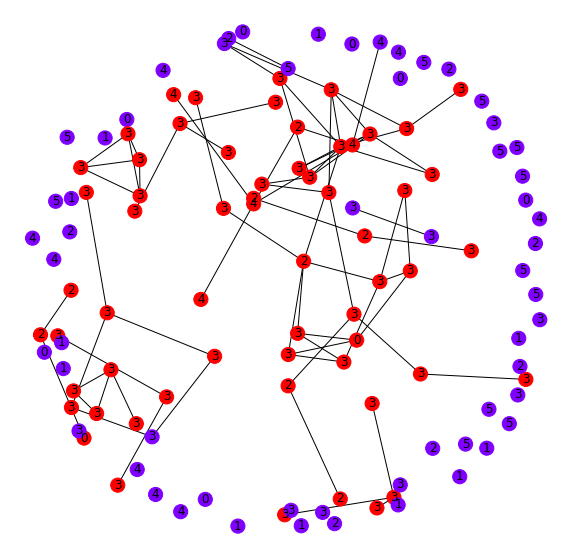Figure 10. Graph structure of new ROI nodes and their nearest neighbors in the graph (red) along with a random sample of nodes (blue). Node labels indicate class.

#### Train GCN with node features

Finally, we train our full model using the word-counts as initial node features. This results in accuracy >70%, which is significantly better than the prior best score of 57%. The graph structure of the citation network clearly adds substantial lift.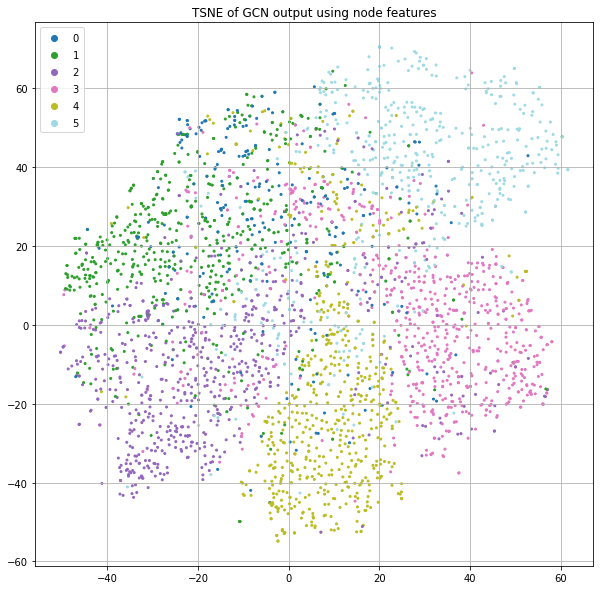Figure 11. t-SNE of GCN output using node features as input. Color indicates class label.

We see that the output in Figure 11 has strong class separation and a “spoke” like structure. Let's visualize the same plot, but color-code by the loss value (Figure 12).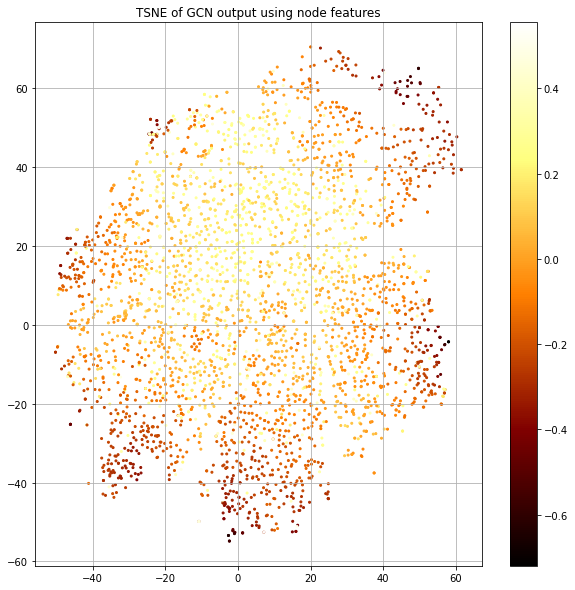Figure 12. t-SNE of GCN output using node features as input. Color indicates (log of) loss value.

The “spokes” have the lowest loss and correspond to particular classes and there's a blending area between spokes where the loss goes up. We also see the center corresponding to uncertainty.

Another interesting observation is that we do not see the highly defined “legs” that characterized the “no features” model in Figure 8. Let's highlight on this plot the same “leg” nodes that we previously deep-dived (Figure 13).Figure 13. t-SNE of GCN output using node features as input. Red points indicate Region of Interest (ROI)

We see here that these points are no longer in a highly-structured band, but are rather spread across the region corresponding to the publications of class 3. In one case, you'll notice a node has moved firmly into the yellow neighborhood with class 4 (idx=3153). Let's look at the new neighbors of this point in the embedding space.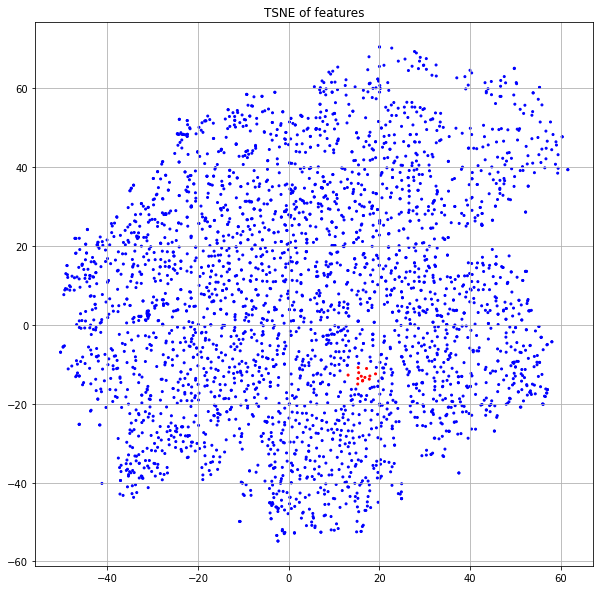Figure 14. t-SNE of GCN output using node features as input. Red points indicate Region of Interest (ROI) around point that moved communities.
>>> # Label of moved/isolated point
>>> label
tensor(4)

>>> # Labels of original "leg" points
>>> label[idx_roi]
tensor([3, 2, 2, 3, 2, 3, 3, 3, 3, 3, 3, 2, 3, 3, 3, 3, 3, 3, 3, 3, 4])

>>> # Labels of new neighborhood of moved/isolated point
>>> label[idx_roi_wf]
tensor([4, 2, 4, 0, 5, 4, 4, 4, 4, 4, 4, 4, 4])


We see that of the original points, 3153 is the only one with label=4. But now, it's new neighborhood has 4 as the dominate label. The node features have allowed the model to recognize this node as being different and put it in a more appropriate neighborhood.

Now let's look at the graph structure of both the new and old embedding neighborhoods to understand their inter-connections (Figure 15).Figure 15. Graph structure of original community and new community of the moved/isolated point. Teal = the node that moved and defines the new community; Blue = nodes shared by both communities; Red = nodes in original community only; Pink = nodes in new community only. Node labels indicate class.

The first thing we notice is that they're all mixed together. The teal node is the one that moved out of the neighborhood once we added the features and was used to define the new neighborhood. The nodes in the original “leg” neighborhood (red) have a dominate class of 3. Nodes in the new neighborhood (pink) have a dominate class of 4. The nodes that are in both neighborhoods (blue) show those that tie these two communities together by linking nodes of different label.

The model is able to use the features to separate a connected set of nodes into two separate community substructures that share connection through a handful of shared nodes, but have different dominating labels within.

## Conclusion

These experiments have shown how node embeddings change as we selectively control the information available to the model. We learned that even when we withhold node features/labels and randomly initialize model parameters, there is still structure in the node embeddings because of the tendency of the GCN computation to put nodes with similar neighborhoods in similar places (Fig. 2). There is a connection here with the Weisfeiler-Lehman algorithm, where the GCN replaces the hash function with a neural network layer for mapping the aggregated neighborhood vector.

Next, we saw that by using informative node features rather than random values, we could get further structure in the embedding space despite still having random model parameters and no training (Fig. 5). This is because we provided the model with an additional “similarity” signal by initializing the node embeddings with their features, therefore putting papers with similar words close together from the start.

When optimizing the model with no feature information for classification, we saw that highly structured bands formed that represented densely inter-connected communities of papers (Fig. 6). In cases that these nodes had sufficient training labels, we also saw strong predictive performance, but in cases where there were few labels, we merely saw structure but poor predictive performance. There was also a large mass of unstructured points with few connections or labels and these had poor classification performance.

However, the addition of node features into the training process transformed this landscape (Fig. 11). The output was less obviously impacted by shared neighborhoods as the “legs” disappeared and instead, well-separated “spokes” of homogenous labels formed. This showed that even though a community may be tightly connected, the model was able to adapt its parameters to use the feature information to further segment these communities for significantly improved classification performance. Whereas the Logistic Regression and Random Forest models were able to use the features of a paper to determine its class, our GCN model was able to also leverage the features of all of its citation network as well, and this improved accuracy from 57% to 70%.

1. Wang, Minjie, et al. “Deep Graph Library: A Graph-Centric, Highly-Performant Package for Graph Neural Networks.” ArXiv:1909.01315 [Cs, Stat], Aug. 2020. arXiv.org, http://arxiv.org/abs/1909.01315.

2. Kipf, Thomas N., and Max Welling. “Semi-Supervised Classification with Graph Convolutional Networks.” ArXiv:1609.02907 [Cs, Stat], Feb. 2017. arXiv.org, http://arxiv.org/abs/1609.02907.

3. Douglas, B. L. “The Weisfeiler-Lehman Method and Graph Isomorphism Testing.” ArXiv:1101.5211 [Math], Jan. 2011. arXiv.org, http://arxiv.org/abs/1101.5211.